# Tools to Format Numbers for Publication

Format numbers and plots for publication; includes the removal of leading zeros, standardization of number of digits, addition of affixes, and a p-value formatter. These tools combine the functionality of several 'base' functions such as 'paste()', 'format()', and 'sprintf()' into specific use case functions that are named in a way that is consistent with usage, making their names easy to remember and easy to deploy.

# numform

numform contains tools to assist in the formatting of numbers and plots for publication. Tools include the removal of leading zeros, standardization of number of digits, addition of affixes, and a p-value formatter. These tools combine the functionality of several 'base' functions such as `paste()`, `format()`, and `sprintf()` into specific use case functions that are named in a way that is consistent with usage, making their names easy to remember and easy to deploy.

# Installation

Download the zip ball or tar ball, decompress and run `R CMD INSTALL` on it, or use the pacman package to install the development version:

``````if (!require("pacman")) install.packages("pacman")
``````

# Contact

You are welcome to:

# Available Functions

Below is a table of available numform functions. Note that `f_` is read as "format" whereas `fv_` is read as "format vector". The former formats individual values in the vector while the latter uses the vector to compute a calculation on each of the values and then formats them. Additionally, all numform `f_` functions have a closure, function retuning, version that is prefixed with an additional `f` (read "format function"). For example, `f_num` has `ff_num` which has the same arguments but returns a function instead. This is useful for passing in to ggplot2 `scale_x/y_type` functions (see Plotting for usage).

 alignment f_byte f_latitude f_peta f_wrap as_factor f_celcius f_list f_pp f_year collapse f_comma f_list_amp f_prefix f_yotta constant_months f_data f_logical f_prop2percent f_zetta constant_months_abbreviation f_data_abbreviation f_longitude f_pval fv_num_percent constant_quarters f_date f_mean_sd f_quarter fv_percent constant_weekdays f_degree f_mega f_replace fv_percent_diff constant_weekdays_abbreviation f_denom f_mills f_response fv_percent_diff_fixed_relative f_12_hour f_dollar f_month f_sign fv_percent_lead f_abbreviation f_exa f_month_abbreviation f_state fv_percent_lead_fixed_relative f_affirm f_fahrenheit f_month_name f_suffix fv_runs f_affix f_giga f_num f_tera glue f_bills f_interval f_num_percent f_thous highlight_cells f_bin f_interval_right f_ordinal f_title f_bin_right f_interval_text f_pad_zero f_weekday f_bin_text f_interval_text_right f_parenthesis f_weekday_abbreviation f_bin_text_right f_kilo f_percent f_weekday_name

Available Formatting Functions

# Demonstration

``````if (!require("pacman")) install.packages("pacman")
``````

## Numbers

``````f_num(c(0.0, 0, .2, -00.02, 1.122222, pi, "A"))
``````

## Abbreviated Numbers

``````f_thous(1234)

##  "1K"

f_thous(12345)

##  "12K"

f_thous(123456)

##  "123K"

f_mills(1234567)

##  "1M"

f_mills(12345678)

##  "12M"

f_mills(123456789)

##  "123M"

f_bills(1234567891)

##  "1B"

f_bills(12345678912)

##  "12B"

f_bills(123456789123)

##  "123B"
``````

...or auto-detect:

``````f_denom(1234)

##  "1K"

f_denom(12345)

##  "12K"

f_denom(123456)

##  "123K"

f_denom(1234567)

##  "1M"

f_denom(12345678)

##  "12M"

f_denom(123456789)

##  "123M"

f_denom(1234567891)

##  "1B"

f_denom(12345678912)

##  "12B"

f_denom(123456789123)

##  "123B"
``````

## Commas

``````f_comma(c(1234.12345, 1234567890, .000034034, 123000000000, -1234567))

##  "1,234.123"       "1,234,567,890"   ".000034034"      "123,000,000,000"
##  "-1,234,567"
``````

## Percents

``````f_percent(c(30, 33.45, .1), 1)

##  "30.0%" "33.5%" ".1%"

f_percent(c(0.0, 0, .2, -00.02, 1.122222, pi))

##  ".0%"  ".0%"  ".2%"  "-.0%" "1.1%" "3.1%"

f_prop2percent(c(.30, 1, 1.01, .33, .222, .01))

##  "30.0%"  "100.0%" "101.0%" "33.0%"  "22.2%"  "1.0%"
``````

## Dollars

``````f_dollar(c(0, 30, 33.45, .1))

##  "\$0.00"  "\$30.00" "\$33.45" "\$0.10"

f_dollar(c(0.0, 0, .2, -00.02, 1122222, pi)) %>%
f_comma()

##  "\$0.00"         "\$0.00"         "\$0.20"         "\$-.02"
##  "\$1,122,222.00" "\$3.14"
``````

Sometimes one wants to lop off digits of money in order to see the important digits, the real story. The `f_denom` family of functions can do job.

``````f_denom(c(12345267, 98765433, 658493021), prefix = '\$')

##  "\$ 12M" "\$ 99M" "\$658M"

f_denom(c(12345267, 98765433, 658493021), relative = 1, prefix = '\$')

##  "\$ 12.3M" "\$ 98.8M" "\$658.5M"
``````

## Tables

Notice the use of the `alignment` function to detect the column alignment.

``````pacman::p_load(dplyr, pander)

set.seed(10)
dat <- data_frame(
Team = rep(c("West Coast", "East Coast"), each = 4),
Year = rep(2012:2015, 2),
YearStart = round(rnorm(8, 2e6, 1e6) + sample(1:10/100, 8, TRUE), 2),
Won = round(rnorm(8, 4e5, 2e5) + sample(1:10/100, 8, TRUE), 2),
Lost = round(rnorm(8, 4.4e5, 2e5) + sample(1:10/100, 8, TRUE), 2),
WinLossRate = Won/Lost,
PropWon = Won/YearStart,
PropLost = Lost/YearStart
)

dat %>%
group_by(Team) %>%
mutate(
`%&Delta;WinLoss` = fv_percent_diff(WinLossRate, 0),
`&Delta;WinLoss` = f_sign(Won - Lost, '<b>+</b>', '<b>&ndash;</b>')

) %>%
ungroup() %>%
mutate_at(vars(Won:Lost), .funs = ff_denom(relative = -1, prefix = '\$')) %>%
mutate_at(vars(PropWon, PropLost), .funs = ff_prop2percent(digits = 0)) %>%
mutate(
YearStart = f_denom(YearStart, 1, prefix = '\$'),
Team = fv_runs(Team),
WinLossRate = f_num(WinLossRate, 1)
) %>%
data.frame(stringsAsFactors = FALSE, check.names = FALSE) %>%
pander::pander(split.tables = Inf, justify = alignment(.))
``````
Team Year YearStart Won Lost WinLossRate PropWon PropLost %ΔWinLoss ΔWinLoss
West Coast 2012 \$2.0M \$350K \$190K 1.9 17% 9% 0% +
2013 \$1.8M \$600K \$370K 1.6 33% 20% -13% +
2014 \$ .6M \$550K \$300K 1.8 87% 48% 11% +
2015 \$1.4M \$420K \$270K 1.6 30% 19% -13% +
East Coast 2012 \$2.3M \$210K \$420K .5 9% 18% 0%
2013 \$2.4M \$360K \$390K .9 15% 16% 86%
2014 \$ .8M \$590K \$ 70K 8.4 74% 9% 811% +
2015 \$1.6M \$500K \$420K 1.2 30% 26% -86% +
``````pacman::p_load(dplyr, pander)

data_frame(
Event = c('freezing water', 'room temp', 'body temp', 'steak\'s done', 'hamburger\'s done', 'boiling water', 'sun surface', 'lighting'),
F = c(32, 70, 98.6, 145, 160, 212, 9941, 50000)
) %>%
mutate(
Event = f_title(Event),
C = (F - 32) * (5/9)
) %>%
mutate(
F = f_degree(F, measure = 'F', type = 'string'),
C = f_degree(C, measure = 'C', type = 'string', zero = '0.0')
)  %>%
data.frame(stringsAsFactors = FALSE, check.names = FALSE) %>%
pander::pander(split.tables = Inf, justify = alignment(.))
``````
Event F C
Freezing Water 32.0°F 0.0°C
Room Temp 70.0°F 21.1°C
Body Temp 98.6°F 37.0°C
Steak's Done 145.0°F 62.8°C
Hamburger's Done 160.0°F 71.1°C
Boiling Water 212.0°F 100.0°C
Sun Surface 9941.0°F 5505.0°C
Lighting 50000.0°F 27760.0°C
``````if (!require("pacman")) install.packages("pacman")

set.seed(11)
data_frame(
date = sample(seq(as.Date("1990/1/1"), by = "day", length.out = 2e4), 12)
) %>%
mutate(
year_4 = f_year(date, 4),
year_2 = f_year(date, 2),
quarter = f_quarter(date),
month_name = f_month_name(date) %>%
as_factor(),
month_abbreviation = f_month_abbreviation(date) %>%
as_factor(),
month_short = f_month(date),
weekday_name = f_weekday_name(date),
weekday_abbreviation = f_weekday_abbreviation(date),
weekday_short = f_weekday(date),
weekday_short_distinct = f_weekday(date, distinct = TRUE)
) %>%
data.frame(stringsAsFactors = FALSE, check.names = FALSE) %>%
pander::pander(split.tables = Inf, justify = alignment(.))
``````
date year_4 year_2 quarter month_name month_abbreviation month_short weekday_name weekday_abbreviation weekday_short weekday_short_distinct
2005-03-07 2005 05 Q1 March Mar M Monday Mon M M
1990-01-11 1990 90 Q1 January Jan J Thursday Thu T Th
2017-12-16 2017 17 Q4 December Dec D Saturday Sat S S
1990-10-08 1990 90 Q4 October Oct O Monday Mon M M
1993-07-17 1993 93 Q3 July Jul J Saturday Sat S S
2042-04-10 2042 42 Q2 April Apr A Thursday Thu T Th
1994-09-26 1994 94 Q3 September Sep S Monday Mon M M
2005-11-15 2005 05 Q4 November Nov N Tuesday Tue T T
2038-03-16 2038 38 Q1 March Mar M Tuesday Tue T T
1996-09-29 1996 96 Q3 September Sep S Sunday Sun S Su
1999-08-02 1999 99 Q3 August Aug A Monday Mon M M
2014-02-14 2014 14 Q1 February Feb F Friday Fri F F

## Plotting

``````library(tidyverse); library(viridis)

set.seed(10)
data_frame(
revenue = rnorm(10000, 500000, 50000),
date = sample(seq(as.Date('1999/01/01'), as.Date('2000/01/01'), by="day"), 10000, TRUE),
site = sample(paste("Site", 1:5), 10000, TRUE)
) %>%
mutate(
dollar = f_comma(f_dollar(revenue, digits = -3)),
thous = f_denom(revenue),
thous_dollars = f_denom(revenue, prefix = '\$'),
abb_month = f_month(date),
abb_week = as_factor(f_weekday(date, distinct = TRUE))
) %>%
group_by(site, abb_week) %>%
mutate(revenue = {if(sample(0:1, 1) == 0) `-` else `+`}(revenue, sample(1e2:1e5, 1))) %>%
ungroup() %T>%
print() %>%
ggplot(aes(abb_week, revenue)) +
geom_jitter(width = .2, height = 0, alpha = .2, aes(color = revenue)) +
scale_y_continuous(label = ff_denom(prefix = '\$'))+
facet_wrap(~site) +
theme_bw() +
scale_color_viridis() +
theme(
strip.text.x = element_text(hjust = 0, color = 'grey45'),
strip.background = element_rect(fill = NA, color = NA),
panel.border = element_rect(fill = NA, color = 'grey75'),
panel.grid = element_line(linetype = 'dotted'),
axis.ticks = element_line(color = 'grey55'),
axis.text = element_text(color = 'grey55'),
axis.title.x = element_text(color = 'grey55', margin = margin(t = 10)),
axis.title.y = element_text(color = 'grey55', angle = 0, margin = margin(r = 10)),
legend.position = 'none'
) +
labs(
x = 'Day of Week',
y = 'Revenue',
title = 'Site Revenue by Day of Week',
subtitle = f_wrap(c(
'This faceted dot plot shows the distribution of revenues within sites',
'across days of the week.  Notice the consistently increasing revenues for',
'Site 2 across the week.'
), width = 85, collapse = TRUE)
)

## # A tibble: 10,000 x 8
##    revenue date       site   dollar thous thous_dollars abb_month abb_week
##      <dbl> <date>     <chr>  <chr>  <chr> <chr>         <chr>     <fct>
##  1 591213. 1999-11-29 Site 1 \$501,~ 501K  \$501K         N         M
##  2 468126. 1999-07-07 Site 4 \$491,~ 491K  \$491K         J         W
##  3 376229. 1999-08-06 Site 2 \$431,~ 431K  \$431K         A         F
##  4 537861. 1999-05-04 Site 3 \$470,~ 470K  \$470K         M         T
##  5 462758. 1999-07-08 Site 4 \$515,~ 515K  \$515K         J         Th
##  6 553879. 1999-07-22 Site 2 \$519,~ 519K  \$519K         J         Th
##  7 473985. 1999-05-20 Site 2 \$440,~ 440K  \$440K         M         Th
##  8 480609. 1999-05-28 Site 5 \$482,~ 482K  \$482K         M         F
##  9 363462. 1999-01-15 Site 2 \$419,~ 419K  \$419K         J         F
## 10 406613. 1999-08-19 Site 3 \$487,~ 487K  \$487K         A         Th
## # ... with 9,990 more rows
``````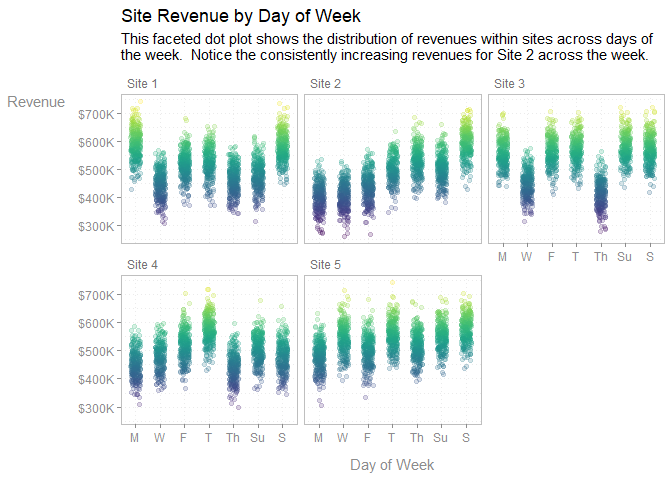``````library(tidyverse); library(viridis)

set.seed(10)
dat <- data_frame(
revenue = rnorm(144, 500000, 10000),
date = seq(as.Date('2005/01/01'), as.Date('2016/12/01'), by="month")
) %>%
mutate(
quarter = f_quarter(date),
year = f_year(date, 4)
) %>%
group_by(year, quarter) %>%
summarize(revenue = sum(revenue)) %>%
ungroup() %>%
mutate(quarter = as.integer(gsub('Q', '', quarter)))

year_average <- dat %>%
group_by(year) %>%
summarize(revenue = mean(revenue)) %>%
mutate(x1 = .8, x2 = 4.2)

dat %>%
ggplot(aes(quarter, revenue, group = year)) +
geom_segment(
linetype = 'dashed',
data = year_average, color = 'grey70', size = 1,
aes(x = x1, y = revenue, xend = x2, yend = revenue)
) +
geom_line(size = .85, color = '#009ACD') +
geom_point(size = 1.5, color = '#009ACD') +
facet_wrap(~year, nrow = 2)  +
scale_y_continuous(label = ff_denom(relative = 2)) +
scale_x_continuous(breaks = 1:4, label = f_quarter) +
theme_bw() +
theme(
strip.text.x = element_text(hjust = 0, color = 'grey45'),
strip.background = element_rect(fill = NA, color = NA),
panel.border = element_rect(fill = NA, color = 'grey75'),
panel.grid.minor = element_blank(),
panel.grid.major = element_line(linetype = 'dotted'),
axis.ticks = element_line(color = 'grey55'),
axis.text = element_text(color = 'grey55'),
axis.title.x = element_text(color = 'grey55', margin = margin(t = 10)),
axis.title.y = element_text(color = 'grey55', angle = 0, margin = margin(r = 10)),
legend.position = 'none'
) +
labs(
x = 'Quarter',
y = 'Revenue (\$)',
title = 'Quarterly Revenue Across Years',
subtitle = f_wrap(c(
'This faceted line plot shows the change in quarterly revenue across',
'years.'
), width = 85, collapse = TRUE)
)
``````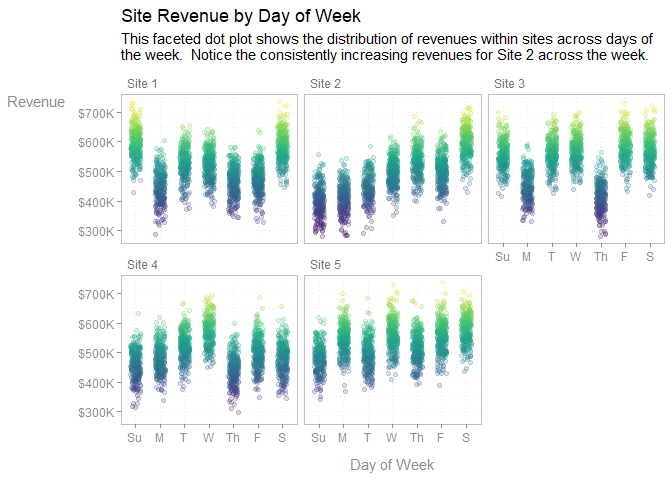``````library(tidyverse); library(gridExtra)

set.seed(10)
dat <- data_frame(
level = c("not_involved", "somewhat_involved_single_group",
"somewhat_involved_multiple_groups", "very_involved_one_group",
"very_involved_multiple_groups"
),
n = sample(1:10, length(level))
) %>%
mutate(
level = factor(level, levels = unique(level)),
`%` = n/sum(n)
)

gridExtra::grid.arrange(

gridExtra::arrangeGrob(

dat %>%
ggplot(aes(level, `%`)) +
geom_col() +
labs(title = 'Very Sad', y = NULL) +
theme(
axis.text = element_text(size = 7),
title = element_text(size = 9)
),

dat %>%
ggplot(aes(level, `%`)) +
geom_col() +
scale_x_discrete(labels = function(x) f_replace(x, '_', '\n')) +
scale_y_continuous(labels = ff_prop2percent(digits = 0))  +
labs(title = 'Underscore Split (Readable)', y = NULL) +
theme(
axis.text = element_text(size = 7),
title = element_text(size = 9)
),

ncol = 2

),
gridExtra::arrangeGrob(

dat %>%
ggplot(aes(level, `%`)) +
geom_col() +
scale_x_discrete(labels = function(x) f_title(f_replace(x))) +
scale_y_continuous(labels = ff_prop2percent(digits = 0))  +
labs(title = 'Underscore Replaced & Title (Capitalized Sadness)', y = NULL) +
theme(
axis.text = element_text(size = 7),
title = element_text(size = 9)
),

dat %>%
ggplot(aes(level, `%`)) +
geom_col() +
scale_x_discrete(labels = function(x) f_wrap(f_title(f_replace(x)))) +
scale_y_continuous(labels = ff_prop2percent(digits = 0))  +
labs(title = 'Underscore Replaced, Title, & Wrapped (Happy)', y = NULL) +
theme(
axis.text = element_text(size = 7),
title = element_text(size = 9)
),

ncol = 2

), ncol = 1

)
``````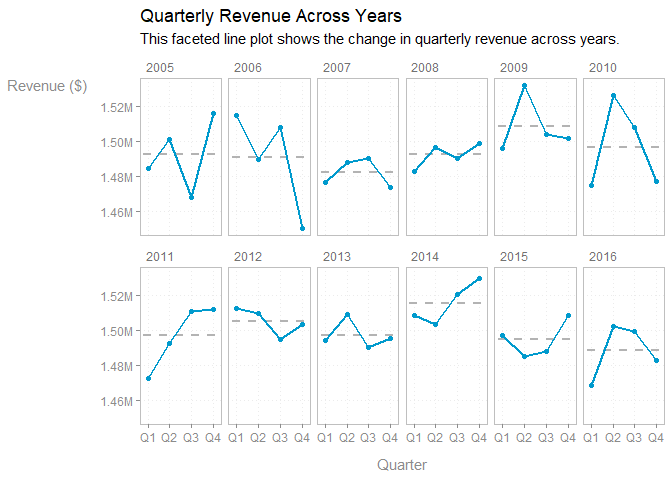``````set.seed(10)
dat <- data_frame(
state = sample(state.name, 10),
value = sample(10:20, 10) ^ (7),
cols = sample(colors()[1:150], 10)
) %>%
arrange(desc(value)) %>%
mutate(state = factor(state, levels = unique(state)))

dat %>%
ggplot(aes(state, value, fill = cols)) +
geom_col() +
scale_x_discrete(labels = f_state) +
scale_fill_identity() +
scale_y_continuous(labels = ff_denom(prefix = '\$'), expand = c(0, 0),
limits = c(0, max(dat\$value) * 1.05)
) +
theme_minimal() +
theme(
panel.grid.major.x = element_blank(),
axis.title.y = element_text(angle = 0)
) +
labs(x = 'State', y = 'Cash\nFlow',
title = f_title("look at how professional i look"),
subtitle = 'Subtitles: For that extra professional look.'
)
``````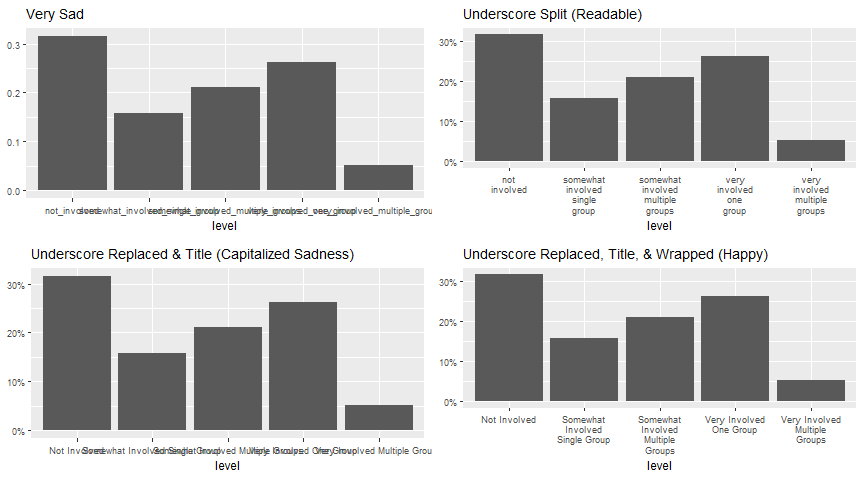``````library(tidyverse); library(viridis)

data_frame(
Event = c('freezing water', 'room temp', 'body temp', 'steak\'s done', 'hamburger\'s done', 'boiling water'),
F = c(32, 70, 98.6, 145, 160, 212)
) %>%
mutate(
C = (F - 32) * (5/9),
Event = f_title(Event),
Event = factor(Event, levels = unique(Event))
) %>%
ggplot(aes(Event, F, fill = F)) +
geom_col() +
geom_text(aes(y = F + 4, label = f_fahrenheit(F, digits = 1, type = 'text')), parse = TRUE, color = 'grey60') +
scale_y_continuous(
labels = f_fahrenheit, limits = c(0, 220), expand = c(0, 0),
sec.axis = sec_axis(trans = ~(. - 32) * (5/9), labels = f_celcius, name = f_celcius(prefix = 'Temperature ', type = 'title'))
) +
scale_x_discrete(labels = ff_replace(pattern = ' ', replacement = '\n')) +
scale_fill_viridis(option =  "magma", labels = f_fahrenheit, name = NULL) +
theme_bw() +
labs(
y = f_fahrenheit(prefix = 'Temperature ', type = 'title'),
title = f_fahrenheit(prefix = 'Temperature of Common Events ', type = 'title')
) +
theme(
axis.ticks.x = element_blank(),
panel.border = element_rect(fill = NA, color = 'grey80'),
panel.grid.minor.x = element_blank(),
panel.grid.major.x = element_blank()
)
``````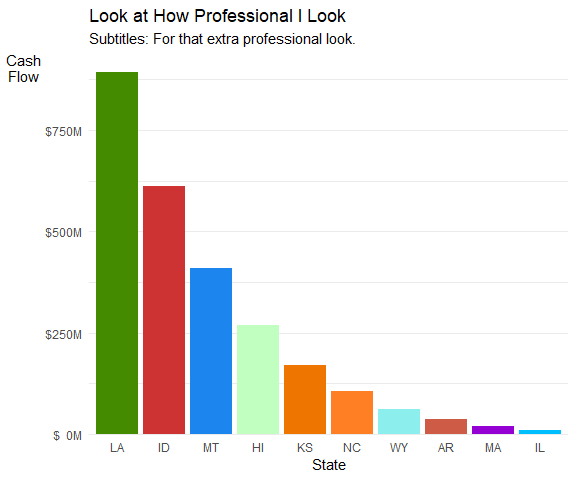``````library(tidyverse); library(maps)

world <- map_data(map="world")

ggplot(world, aes(map_id = region, x = long, y = lat)) +
geom_map(map = world, aes(map_id = region), fill = "grey40", colour = "grey70", size = 0.25) +
scale_y_continuous(labels = f_latitude) +
scale_x_continuous(labels = f_longitude)
``````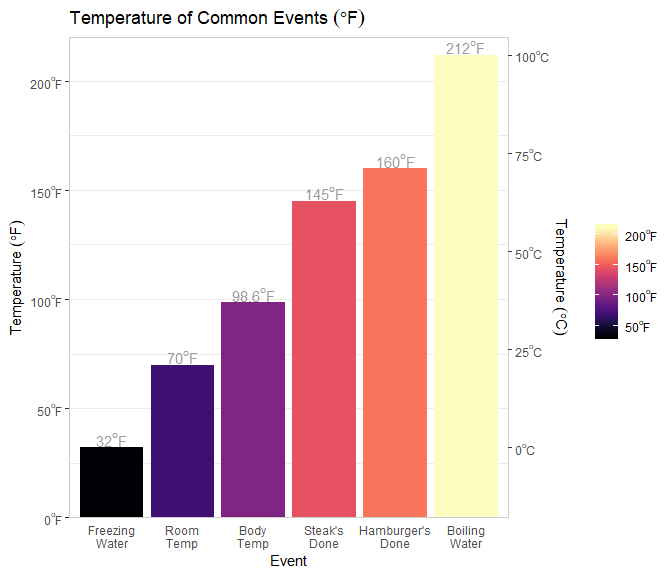``````mtcars %>%
mutate(mpg2 = cut(mpg, 10, right = FALSE)) %>%
ggplot(aes(mpg2)) +
geom_bar(fill = '#33A1DE') +
scale_x_discrete(labels = function(x) f_wrap(f_bin_text_right(x, l = 'up to'), width = 8)) +
scale_y_continuous(breaks = seq(0, 14, by = 2), limits = c(0, 7)) +
theme_minimal() +
theme(
panel.grid.major.x = element_blank(),
axis.text.x = element_text(size = 14, margin = margin(t = -12)),
axis.text.y = element_text(size = 14),
plot.title = element_text(hjust = .5)
) +
labs(title = 'Histogram', x = NULL, y = NULL)
``````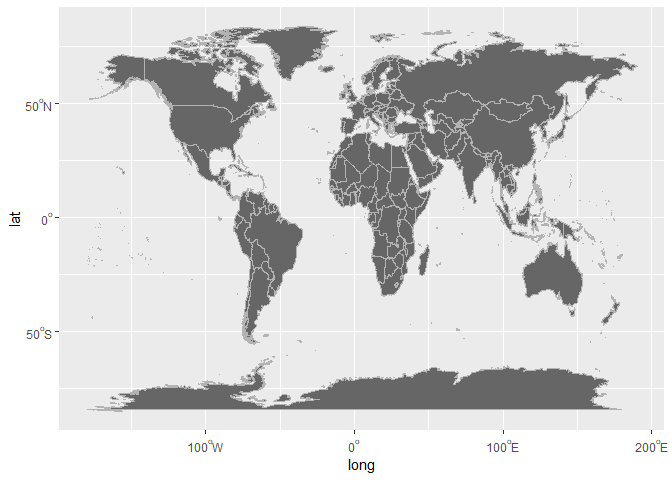``````dat <- data_frame(
Value = c(111, 2345, 34567, 456789, 1000001, 1000000001),
Time = 1:6
)

gridExtra::grid.arrange(

ggplot(dat, aes(Time, Value)) +
geom_line() +
scale_y_continuous(labels = ff_denom( prefix = '\$')) +
labs(title = "Single Denominational Unit"),

ggplot(dat, aes(Time, Value)) +
geom_line() +
scale_y_continuous(
labels = ff_denom(mix.denom = TRUE, prefix = '\$', pad.char = '')
) +
labs(title = "Mixed Denominational Unit"),

ncol = 2
)
``````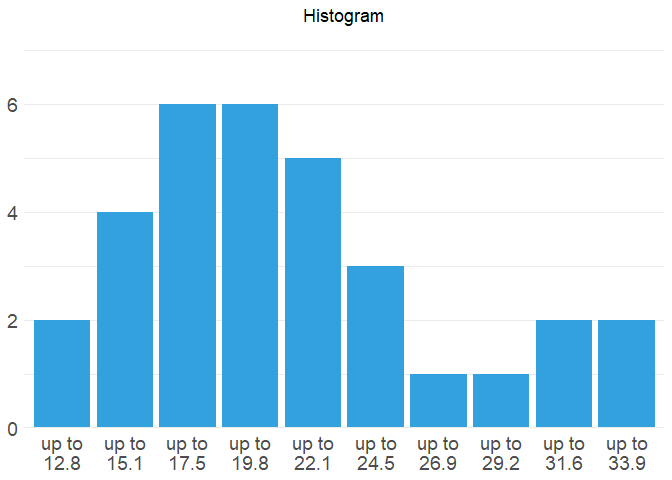## Modeling

We can see its use in actual model reporting as well:

``````mod1 <- t.test(1:10, y = c(7:20))

sprintf(
"t = %s (%s)",
f_num(mod1\$statistic),
f_pval(mod1\$p.value)
)

##  "t = -5.4 (p < .05)"

mod2 <- t.test(1:10, y = c(7:20, 200))

sprintf(
"t = %s (%s)",
f_num(mod2\$statistic, 2),
f_pval(mod2\$p.value, digits = 2)
)

##  "t = -1.63 (p = .12)"
``````

We can build a function to report model statistics:

``````report <- function(mod, stat = NULL, digits = c(0, 2, 2)) {

stat <- if (is.null(stat)) stat <- names(mod[["statistic"]])
sprintf(
"%s(%s) = %s, %s",
gsub('X-squared', '&Chi;<sup>2</sup>', stat),
paste(f_num(mod[["parameter"]], digits), collapse = ", "),
f_num(mod[["statistic"]], digits),
f_pval(mod[["p.value"]], digits = digits)
)

}

report(mod1)

##  "t(22) = -5.43, p < .05"

report(oneway.test(count ~ spray, InsectSprays))

##  "F(5, 30) = 36.07, p < .05"

report(chisq.test(matrix(c(12, 5, 7, 7), ncol = 2)))

##  "&Chi;<sup>2</sup>(1) = .64, p = .42"
``````

This enables in-text usage as well. First set up the models in a code chunk:

``````mymod <- oneway.test(count ~ spray, InsectSprays)
mymod2 <- chisq.test(matrix(c(12, 5, 7, 7), ncol = 2))
``````

And then use ``r report(mymod)`` resulting in a report that looks like this: F(5, 30) = 36.07, p < .05. For Χ2 using proper HTML leads to Χ2(1) = .64, p = .42.

# NEWS

## Versioning

Releases will be numbered with the following semantic versioning format:

..

And constructed with the following guidelines:

• Breaking backward compatibility bumps the major (and resets the minor and patch)
• New additions without breaking backward compatibility bumps the minor (and resets the patch)
• Bug fixes and misc changes bumps the patch

## numform 0.4.1 - 0.5.0

BUG FIXES

• Functions that enable the argument `less.than.replace = TRUE` would result in the wrong solution if the input value was negative. This has been corrected.

• `f_quarter` didn't respect the `space` or `max` arguments for some methods.

• `alignment` failed to align correctly if there were leading spaces.

NEW FEATURES

• `highlight_cells` added as a quick and dirty way to add cell font highlighting.

• The `collapse` and `glue` functions from the glue package are reexported for easy string manipulation.

• `f_bin` (a.k.a. `f_interval`) added for turning `"[3,5)"` form from `cut` into a more human readable for. There are several different forms of `f_bin` so use `?f_bin` to see them all.

• `fv_percent_lead` added to work similar to `fv_percent_diff` but using the formula T_n/T_n-1.

• `fv_percent_lead_fixed_relative` & `fv_percent_diff_fixed_relative` added in order to compute `fv_percent_lead` & `fv_percent_diff` but keeping the comparison year as a constant rather than using the prior year in the vector of elements.

• `f_data` and friends (see `?f_data`) added to convert between data units. For example, move from 10,000,000,000 (Bytes) to 10GB (Gigabytes) instead.

MINOR FEATURES

• `f_denom` family of functions picks up a `less.than.replace` argument to replace values that say zero with a less than sing followed by a one and then the denomination. For example, in `c(2, 1234)` the digit 2 becomes "<1K".

• `f_denom` picks up a `mix.denom` argument to allow for the mixing of denominations. This is useful for tables with a total row that is a denomination higher than the rest of the column.

• `f_pp` added to lower typing on `f_prop2percent(digits = 0)` (a commonly used function and setting) and to also change the default digits value to 0 as is typically used in plotting.

• `constant_weekdays_abbreviation` added to provide an abbreviated form of weekdays.

• `f_month_name` & `f_month_abbreviation` added to convert to full name and 3 letter abbreviated month formats.

• `f_weekday_name` & `f_weekday_abbreviation` added to convert to full name and 3 letter abbreviated weekday formats.

CHANGES

• The argument `less_than_replace` in `f_prop2percent` & `f_percent` has been replaced with `less.than.replace` to make dot case consistent for arguments across the package.

## numform 0.3.1 - 0.4.0

BUG FIXES

• `f_12_hour` did not format correctly if the vector contained missing (`NA`) values; see issue #12.

NEW FEATURES

• `f_year`, `f_12_hour`, `f_month`, and `f_weekday` (methods based functions) did not previously have closure, function retuning, forms (i.e., `ff_` form) making them inconsistent in usage. These functions all have a closure, function returning, form.

• `f_quarter` added for working with business quarters.

• `f_degree` and friends (`f_latitude`, `f_longitude`, `f_fahrenheit`, & `f_celcius`) added for working with degrees in scales and tables.

MINOR FEATURES

• `f_wrap` picks up an `equal.lines` argument to add additional lines to make each element have the same number of new line characters. This is useful for legend spacing.

• `f_wrap` picks up a `collapse` argument to `paste` + `collapse` a vector before processing. This is useful wrapping subtitles or other longer text blocks.

• `f_title` picks up an `upper` and `lower` argument to allow the user to supply a vector of regexes to force to be upper/lower regardless of title case.

## numform 0.0.6 - 0.3.0

NEW FEATURES

• `f_affirm` and `f_logical` added to convert logical and dummy values into yes/No or True/False strings respectively.

• alignment` added to easily align columns in table formatting functions from add-on R packages which may be confused by numform's conversion of numeric to character class, though right table alignment should be maintained.

• `f_date` and `f_12_hour` added to convert dates and times into common in-text form.

• `f_replace` added as a ggplot2 scale `gsub` convenience function. Defaults to replace '_' with ' '.

• `f_title` added as a ggplot2 scale `tools::toTitleCase` convenience function.

• `f_wrap` added as a ggplot2 scale `strwrap` + `paste(collapse =TRUE)` convenience function.

• `f_state` added for converting state names to their abbreviated form for plots.

• `f_year` added as a ggplot2 scale to convert to 2 digit year form convenience function.

• `f_abbreviation` added for converting string names to their abbreviated form.

• constants added for weekdays, months, and month abbreviations in order to make factor conversion of levels easier. See `?constant_months` for more.

MINOR FEATURES

• `f_sign` picks up `negative` and `positive` assignments allowing for more control in format (previously +/- were assigned). This enables the ability to give other characters tailored for document formats such as html, LaTeX, etc.

• `f_prop2percent` and `f_percent` pick up a logical `less_than_replace` argument that replaces values lower than this with a less than sign and the cut point specified by the `digits` argument.

CHANGES

• `f_denom` now returns `x` if the `max` < 1K.

## numform 0.0.1 - 0.0.5

Format numbers for publication; includes the removal of leading zeros, standardization of number of digits, addition of affixes, and a p-value formatter. These tools combine the functionality of several 'base' functions such as paste(), format(), and sprintf() into specific use case functions that are named in a way that is consistent with usage, making their names easy to remember and easy to deploy.

# Reference manual

install.packages("numform")

0.7.0 by Tyler Rinker, 2 months ago

https://github.com/trinker/numform

Report a bug at https://github.com/trinker/numform/issues

Browse source code at https://github.com/cran/numform

Authors: Tyler Rinker [aut, cre]

Documentation:   PDF Manual